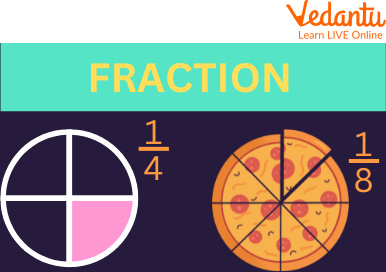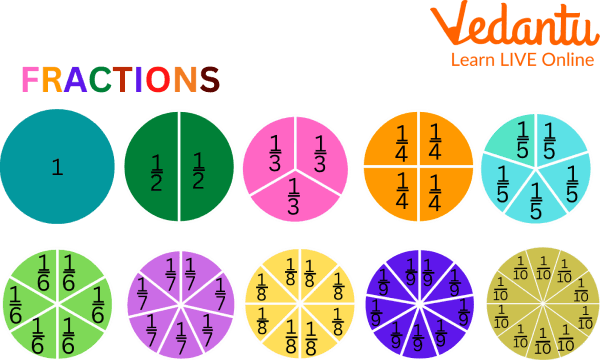Courses
Courses for Kids
Free study material
Free LIVE classes
More

# Fraction NumbersLIVE
Join Vedantu’s FREE Mastercalss

## Introduction to the Fraction Numbers

Fraction is a very important part of our everyday life. We use it in our daily life without knowing it, like if we have a cake and we cut it into slices, while following a recipe, while dividing a pizza, and in many other actions.

But if you don't know anything about fractions then don't worry. In this article we are going to learn what fractional numbers are, will also see some fractional number examples, properties of fractional numbers, and many other interesting things which are related to fractional numbers. We are going to learn all these things in an enjoyable way so you don't get bored while learning so let's get started.Fraction

## What are Fractional Numbers?

Before coming to the fractional number we need to understand what fraction means.

So a fraction is defined as a part of the whole.

If we take a piece of chocolate from the whole then it's also a fraction like if we say in a whole piece of chocolate there are 8 pieces and if we take one from it then it's \$\dfrac{1}{8}\$ here 1 is the numerator and 8 is the denominator.

The numerator is the part that we take and the denominator is the actual whole number from which we take it.

We use fractional numbers every time when we take a part from the whole.

So with the help of a fractional number, we explain the fraction. That is the total number of parts we had used from the whole.Numerator and Denominator

## Types of Fraction

• Proper fraction- It's a fraction where the numerator is always less than the denominator. Like \$\dfrac{8}{11}\$, \$\dfrac{7}{9}\$, \$\dfrac{6}{9}\$

So in this every numerator is less than the denominator.

• Improper fraction- It's the fraction where the numerator is always more than the denominator. Like \$\dfrac{5}{3}\$, \$\dfrac{7}{5}\$, \$\dfrac{3}{1}\$.

So in this, every numerator is greater than the denominator.

• Mixed fraction- It's a combination type of fraction in which there is a part of an integer and a proper fraction. Like \$5 \dfrac{7}{8}\$, \$6 \dfrac{1}{4}\$,

So in this there is a combination of both integers and the proper fraction.

## Properties of Fraction

• Associative property- It's applied to fraction numbers also. But only in addition and multiplication. Like if we interchange the place of fractional numbers while adding or multiplying the answer remains the same.

• Commutative property- It's applied to fractional numbers too but only in the case of addition and multiplication of fractions.

• Identity property- the identity element for the fractional addition is zero "0", and for fractional multiplication is 1.

• Inverse property- here the inverse of \$\dfrac{a}{b}\$ is \$\dfrac{b}{a}\$, where a and b are non-zero numbers.

• Distributive property- fraction follows the distributive property.Fractions

## Rules for Simplification of Fraction

• First rule says that if we divide the fraction from another fraction then we need to reciprocate the second fraction and then multiply it with the first fraction.

• Second rule is for the multiplication of fractions. So the second rule says that if we multiply two fractional numbers then the numerator and the denominator both are multiplied with the numerator and denominator of the second fraction and after that we simplify the fraction.

• And the third rule is for addition or subtraction of fractional numbers. It says that before adding or subtracting a fractional number we need to make sure that the denominator is equal because the addition or subtraction is possible to fractional numbers if their denominator is the same.

### Solved Example

Q1. In a fruit basket, there are 13 fruits, 5 of which are mango. How can we express the number of mangoes as a fraction?

Ans: \$\dfrac{5}{13}\$

Where 5 is the number of mangoes

And 13 is the total number of fruits which were there in the basket.

Q2. Tanishka spends \$\dfrac{1}{3}\$ of her money her parents give her on a toy and she also spends \$\dfrac{1}{9}\$ of her money on candies. Calculate what fraction of payment will Tanishka spend?

Ans: Find the common denominator

So we will write \$\dfrac{1}{3}\$ as \$\dfrac{3}{9}\$

Now we calculate;

\$\dfrac{3}{9}+\dfrac{1}{9}=\dfrac{4}{9}\$

= \$\dfrac{4}{9}\$

So Tanishka spends \$\dfrac{4}{9}\$ of her money.

Q3. An apple is cut into two parts. You need to write its fraction.

Ans: \$\dfrac{1}{2}\$

### Practice Questions

Q1. It takes Neha \$\dfrac{1}{2}\$ hour to wash, comb her hair and to put on her beautiful clothes, and she takes \$\dfrac{1}{4}\$ hour to have her breakfast. How much time does it take Neha to be ready for her school?

Ans. \$\dfrac{3}{4}\$ of an hour

Q2. Naman ate \$1 \dfrac{1}{4}\$ pizzas and Tanya ate \$1 \dfrac{2}{3}\$ pizzas. How much more pizza did Tanya eat than Naman?

Ans. Tanya ate \$\dfrac{5}{12}\$ of the pizza more than Naman.

## Summary

So in this article we learned about fractions in a very easy and enjoyable way. We learned here about what fractions are, what are the properties of fraction, what the rules of fraction, what are some of its types and we also now understand how we use it in our everyday life without knowing it. We understand that fractions are very important to understand because we use it on a daily basis while taking apart from the whole we are doing fraction. With the help of this article we had cleared our concept related to the fraction.

Last updated date: 23rd Sep 2023
Total views: 92.7k
Views today: 1.92k

## FAQs on Fraction Numbers

1. Who invented fractions?

Simon Steven invented the fractions.

2. Do we use fractions in everyday life?

Fractions are an indispensable part of our life and without even realizing it, we constantly use fractions in our day-to-day tidings. Whether it is \$\dfrac{1}{2}\$ a dozen bananas in your shopping list or baking a yummy chocolate cake with \$\dfrac{3}{4}\$th cup of flour and \$\dfrac{1}{4}\$th cup of milk, everything around us involves fractions.

3. Where do fractions come from?

Egyptians based their numeral system using "base ten," this allowed them to create a way in which numbers could be written. They used hieroglyphics to represent these numbers, but soon the Egyptians faced a slight problem. They needed a way to split food among people. This propelled the idea of fractions.